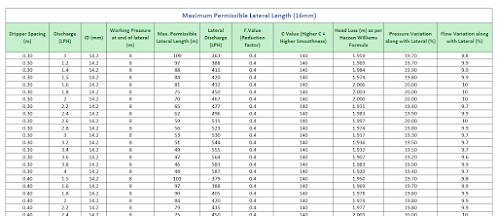# Learn Drip Irrigation System DesignYou can find each and every aspect of Micro Irrigation System design in my blog post.

Drip Irrigation System Design - Basic Aspectshrdpgajjar-​dripirrigation.​blogspot.​com

Basic information on how to design micro irrigation system.

As an example:

### Lateral Length Calculation in Micro Irrigation System

Hi all,

Today I am going to explain an important aspect in micro irrigation system design i.e. Lateral length calculation.

Lateral length affects mainly for deciding submain position in the field. Over run lateral length might be harmful to the crop because water will not distributed in equal manner in the field. Which ultimately results in poor crop production and loss to the farmer.

So lets start on how to calculate maximum permissible lateral length in micro irrigation system.

There are several points to be taken in to consideration for calculation of lateral length which are,

- Lateral outer diameter and inner diameter

- Reduction Factor

- Pressure Drop (m/100 m)

- Pressure Variation along with lateral (%)

- Discharge Variation along with lateral (%)

Pressure Drop can be calculated by the Hazen Williams equation as under,Where,

Flow = Lateral Discharge (lph)

Constant = 140 (hazen williams constant, higher C value = higher smoothness)

Pipe ID = 14.2 mm for 16 mm lateral and 18.2 mm for 20 mm lateral

Reduction Factor = 0.4

Total Head Loss can be calculated with below formula,Pressure Variation along with lateral can be calculated as under,Flow Variation along with lateral can be calculated by,Now as we have come to know that how to calculate maximum permissible lateral length, there are some basic rules to taken in to consideration while calculating length of emitting pipe for its optimum working are as under,

Criteria for optimum lateral length,

1. Total Head Loss should not be more than  2 meter  in lateral or emitting pipe

2. Pressure Variation should not be more than  20%  in lateral

3. Flow Variation should not be more than  10%  in lateral

4. Pressure Loss should not be less than  0.8 m  at the end of the lateral

For ready reference I have created a lateral length table using above mentioned formula.